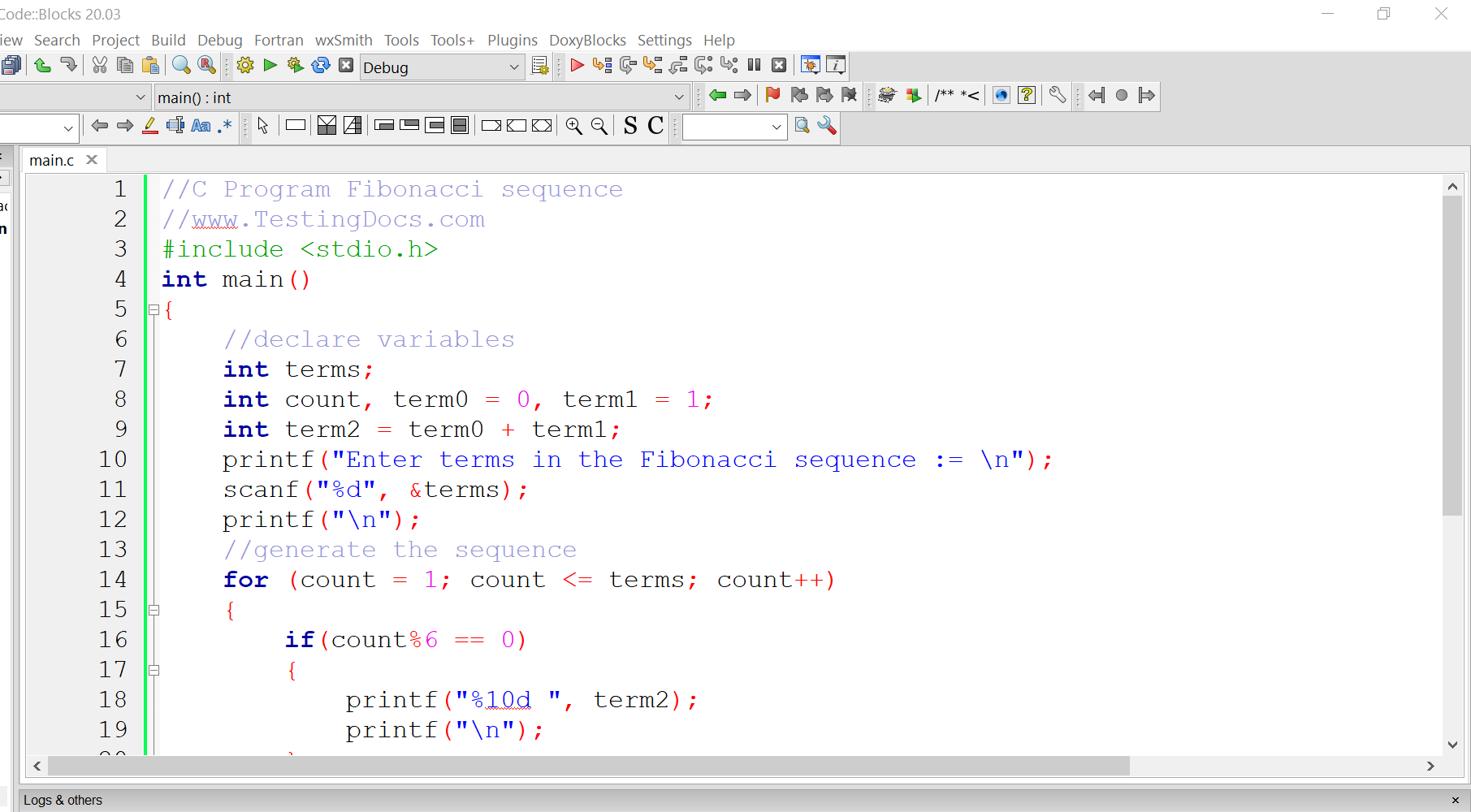# C Program to generate Fibonacci sequence into rows and columns

### Overview

In this post, we will develop C Program to generate Fibonacci sequence into rows and columns as output. We get the Fibonacci sequence nth term by adding the previous two terms in the sequence. i.e Fib(n) = Fib(n-1) + Fib(n-2)

### C Program

```//C Program Fibonacci sequence
//www.TestingDocs.com
#include
int main()
{
//declare variables
int terms;
int count, term0 = 0, term1 = 1;
int term2 = term0 + term1;
printf("Enter terms in the Fibonacci sequence := \n");
scanf("%d", &terms);
printf("\n");
//generate the sequence
for (count = 1; count <= terms; count++)
{
if(count%6 == 0)
{
printf("%10d ", term2);
printf("\n");
}
else
{
printf("%10d ", term2);
}
term2 = term0 + term1;
term0 = term1;
term1 = term2;

}
return 0;
}```

###